Thermodynamics and Propulsion

An ideal thermal radiator is called a black body.'' It has several properties:
1. It has, and absorbs all radiation incident on it.
2. The energy radiated per unit area iswhereis the Stefan-Boltzmann constant,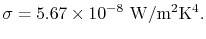The units of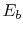are therefore W/m2

The energy of a black body,, is distributed over a range of wavelengths of radiation. We can define, the energy radiated per unit area for a range of wavelengths of width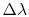. The behavior ofis given in Figure 19.2.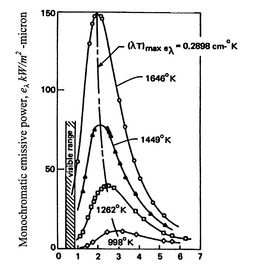The distribution ofvaries with temperature. The quantityat the condition whereis a maximum is given by. As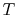increases, the wavelength for maximum energy emission shifts to shorter values. The frequency of the radiation,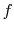, is given by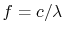so high energy means short wavelengths and high frequency.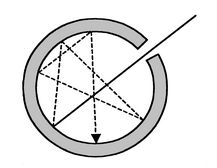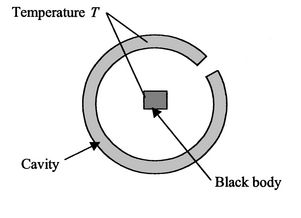A physical realization of a black body is a cavity with a small hole (Figure 19.3). There are many reflections and absorptions. Very few entering photons (light rays) will get out. The inside of the cavity has radiation which is homogeneous and isotropic (the same in any direction, uniform everywhere).

Suppose we put a small black body inside the cavity as seen in Figure 19.4. The cavity and the black body are both at the same temperature.

The radiant energy absorbed by the black body per second and per m2 is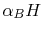, whereis the irradiance, the radiant energy falling on any surface inside the cavity. The radiant energy emitted by the black body is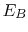. Since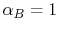for a black body,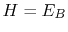. The irradiance within a cavity whose walls are at temperatureis therefore equal to the radiant emittance of a black body at the same temperature and irradiance is a function of temperature only.

UnifiedTP